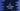# C program to print a right angled triangle using numbers### C program to print right angled triangle using number :

In this C programming tutorial, we will learn how to create a right angled triangle using numbers. Like below :

``````1
1 2
1 2 3
1 2 3 4
1 2 3 4 5
``````
1. The height of the triangle is 5.
2. For line 1 only 1 number is printed. For line 2, 2 numbers are printed etc.
3. All the numbers are printed serially i.e. like 1 2 3 4 5 6…

To solve this problem, we will use two loops. One outer-loop and one inner-loop. The outer-loop will run for ‘height’ number of times, where ‘height’ is the height of the triangle. In the above example, it is 5. The inner-loop will run for outer-loop position number of times. For the first line, outer-loop position is 1 , so the inner loop will run 1 time. For the second line, it will run for 2 times etc. We will use the inner-loop to print the numbers. After the inner loop is completed, one new line will be printed at the end.

Let’s take a look at the program to understand it more clearly :

### C program :

``````#include <stdio.h>

int main()
{
//1
int height;
int i, j;

//2
printf("Enter height of the triangle : ");
scanf("%d", &height);

//3
for (i = 1; i <= height; i++)
{
//4
for (j = 1; j <= i; j++)
{
printf("%d ", j);
}
//5
printf("\n");
}
}``````

### Explanation :

The commented numbers in the above program denotes the step-number below :

1. Create one integer variable height to store the user-input height of the triangle. i,j integers are used in the loop below.
2. Ask the user to enter the height . Save it in variable height
3. Start two for-loops. The outer loop will run for height number of times. It runs for i from 1 to height.
4. The inner-loop will run for i number of times.
5. In the inner-loop, print the values serially.
6. After the inner-loop is completed, print one new-line.

### Sample Output :

``````Enter height of the triangle : 6
1
1 2
1 2 3
1 2 3 4
1 2 3 4 5
1 2 3 4 5 6

Enter height of the triangle : 10
1
1 2
1 2 3
1 2 3 4
1 2 3 4 5
1 2 3 4 5 6
1 2 3 4 5 6 7
1 2 3 4 5 6 7 8
1 2 3 4 5 6 7 8 9
1 2 3 4 5 6 7 8 9 10

``````